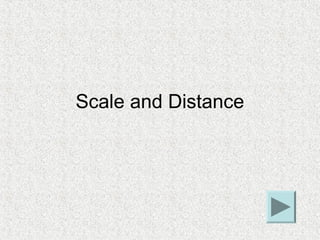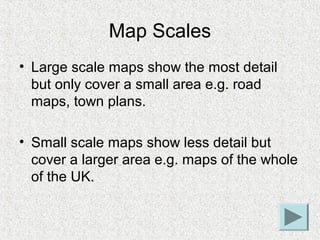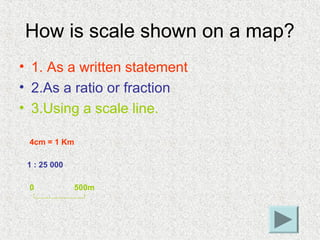Seu SlideShare está sendo baixado. ×

# Scale and distance

Anúncio
Anúncio
Anúncio
Anúncio
Anúncio
Anúncio
Anúncio
Anúncio
Anúncio
Anúncio
AnúncioCarregando em…3
×

1 de 10 Anúncio

# Scale and distance

I do not own this presentation, I cannot remember where I found it. No breach of copyright intended. If you know the owner please notify me.

I do not own this presentation, I cannot remember where I found it. No breach of copyright intended. If you know the owner please notify me.

Anúncio
Anúncio

### Scale and distance

1. 1. Scale and Distance
2. 2. Map Scales • Large scale maps show the most detail but only cover a small area e.g. road maps, town plans. • Small scale maps show less detail but cover a larger area e.g. maps of the whole of the UK.
3. 3. How is scale shown on a map? • 1. As a written statement • 2.As a ratio or fraction • 3.Using a scale line. 4cm = 1 Km 1 : 25 000 0 500m
4. 4. OS Maps • On an OS map the blue grid lines (the eastings and northings) always make up 1Km grid squares. • So these squares will be 4cm long on a 1 : 25000 map but only 2cm long on a 1 : 50 000 map etc. • The scales mean the ratio of the distance on the map with the actual distance in real life. • A 1cm line on a 1 : 50 000 map will be 50 000 cms along the ground (or ½ Kilometre) • Or a 4cm line on a 1 : 25 000 map will be 100 000cms (4 x 25,000) along the ground (or 1 Kilometre)
5. 5. How to measure distances on a map 1. Straight line distances • The shortest distance between two points is sometimes known as the distance ‘as the crow flies’. • This can be measure with a ruler then converted to the correct scale with reference to the scale bar given on the map.
6. 6. Let’s measure the distance between the caravan park and the windmill on this map. 0 500 1Km 2Km As you can see the distance is 2Km.
7. 7. How to measure distances on a map 2. Curved Distances • You may need to measure the distance along a road or river that does not travel in a straight line. • To do this you ideally need a piece of string (or you can use a strip of paper). • You lay the string down to follow the shape then measure the total length before converting back using the scale. • If using paper you need to pivot the paper each time the path changes direction.
8. 8. Now Let’s measure the distance along the railway on this map. 1. Note the points where the direction changes. 2. Measure the distance between each one. 3. Add them up then convert using the scale bar. 0 500 1Km 2Km This would be 7½ Km
9. 9. • Measurement of distances can be used to work out rough areas of features such as woodlands. • Just measure the two main sides, convert the distances using the scale bar then multiply together to give the 0 500m rough area. Burnham Wood is roughly 1500m x 1300m which means it has an area of about 1 950 000m square or 1.95Km square.